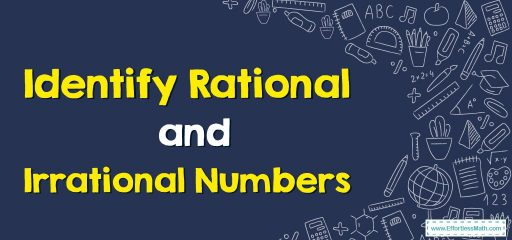# How to Identify Rational and Irrational Numbers?

In this post blog, we teach you the definition of rational and irrational numbers and how to identify them.## A step-by-step guide to rational and irrational numbers

Different types of numbers depend on their properties. For example, rational and irrational numbers are as follows:

### Rational numbers

A rational number is a number in the form $$\frac{p}{q}$$ where $$p$$ and $$q$$ are integers and $$q$$ is not equal to $$0$$.  The set of rational numbers is denoted by $$Q$$.

#### How to identify rational numbers?

Rational numbers can be easily identified with the help of the following properties.

• All integers, whole numbers, natural numbers, and fractions with integers are rational numbers.
• If the decimal form of the number is terminating or repeating, such as $$5.6$$ or $$3.151515$$, we know that they are rational numbers.
• If the decimal numbers seem never-ending or non-repeating, they are called irrational numbers.
• Another way to identify rational numbers is to see if the number can be expressed as $$\frac{p}{q}$$, where $$p$$ and $$q$$ are integers and $$q$$ is not equal to $$0$$.

### Irrational numbers

Irrational numbers are the set of real numbers that cannot be expressed as fractions, $$\frac{p}{q}$$ where $$p$$ and $$q$$ are integers. The denominator $$q$$ is not equal to zero $$(q≠0)$$. The set of irrational numbers is represented by $$Q´$$.

#### How to identify an irrational number?

We know that irrational numbers are just real numbers that cannot be expressed as $$\frac{p}{q}$$, where $$p$$ and $$q$$ are integers and $$q≠0$$. For example, $$\sqrt{5}$$ and $$\sqrt{3}$$, etc. are irrational numbers. On the other hand, numbers that can be represented as $$\frac{p}{q}$$, such that $$p$$ and $$q$$ are integers and $$q≠0$$, are rational numbers.

### Rational and Irrational numbers–Example 1

Determine the rational numbers among the following. $$\sqrt{16},\:\sqrt{3},\:-\frac{4}{5},\:\pi ,\:2.3137134623730860…..$$

Solution: $$\sqrt{16},-\frac{4}{5}$$ are rational numbers.

### What people say about "How to Identify Rational and Irrational Numbers? - Effortless Math: We Help Students Learn to LOVE Mathematics"?

No one replied yet.

X
30% OFF

Limited time only!

Save Over 30%

SAVE $5 It was$16.99 now it is \$11.99